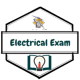# SSC JE electrical paper 2014 Morning shift with explanation |MES Electrical

Ques 1. A lamp having mean spherical candle power of 800 is suspended at a height of 10 m. Calculate the illumination just before the lamp

1. 8000 lux
2. 8 lux
3. 80 lux
4. 800 lux

Illumination in foot-candles on plane normal to axis of light = Candle-power/ (distance)2

E = Icosθ/d2

=  800/102 = 8 lux

Ques 2. Hydrogen is used in large alternator mainly to

1. Reduce eddy current losses
2. Reduce distortion of waveform
3. Cool the machine
4. Strengthen the magnetic field

# Why is hydrogen used for Alternator cooling?

Hydrogen is least expensive, less weight, high thermal conductivity, less density and less viscosity. Less weight, less density & less viscosity attributes to its flow rate. High thermal conductivity helps in better heat exchange. Least expensive helps in balance sheets, more power in less investments.

In order to reduce high temperature of alternator hydrogen gas is used as a coolant. The coolant, Hydrogen gas is allowed to flow in a closed cyclic path around the rotor. Heat exchange takes place and the temperature of hydrogen gas increases, for better cooling of the rotor in next cycle it has to be cooled. Cooling of hydrogen gas is done by passing it through heat exchangers generally constituted with water. Now Hydrogen gas after cooling is allowed to pass through driers ( mainly silica gel which absorbs moisture) and allowed to pass again through the rotor.

Ques 3. Two wires A and B have the same cross-section and are made of the same material. RA = 800Ω and RB = 100Ω. The number of times A is longer than B is

1. 5
2. 6
3. 2
4. 4

None of the options is correct check Explanation

Since they are of the same material they will have the same resistivity.

Therefore their length now

Ra/Rb=la/lb

800/100 = la/lb

la = 8lb

Therefore A is 8 times longer than B

Ques 4. In the circuit shown in the figure, find the transient current i(t) when the switch is closed at t = 0, Assume zero initial condition.1. 50 t e-0.5t
2. 50 t e-5t
3. 100 t e-5t
4. 100 t e-0.5tQues5.  The Ebers-Moll model is applicable to:

1. JFET
2. BJT
3. NMOS transistor
4. UJT

Ebers Moll model is a simple and elegant way of representing the transistor as a circuit model.This model is based on the assumption that base spreading resistance can be neglected. Ebers Moll model is one of the classical models of BJT for small signals. This model is based on interacting diode junctions and is applicable to any transistor operating in active mode

Ques6. A DC voltmeter has a sensitivity of 1000Ω /volt. When it measures half full scale in 100 V range, the current through the voltmeter will be

1. 50 mA
2. 100 mA
3. 1 mA
4. 0.5 mA

Sensitivity of voltmeter (s) = full scale deflection of voltmeter/current passing through voltmeter

Method 1

For 1 volt resistance is 1000 ohm as given in the question.

For 100 volt resitance = 1000 x 100 ohm

And thus full current in the meter = v/r

= (100)/(1000*100)

=1ma

And therefore half full scale 1/2= 0.5ma

Ques 7. A delta-star transformer has a phase to phase voltage transformation ratio of a : 1 [delta phase: star phase]. The line to line voltage ratio of star-delta is given by

1. a/1
2. √3/√a
3. a√3
4. √3/aQues 8. Which of the following motors can be run on A.C as well as D.C supply?

1. Reluctance Motor
2. Universal Motor
3. Repulsion Motor
4. Synchronous Motor

• The universal motor is so named because it is a type of electric motor that can operate on AC or DC power.
• It is a commutated series-wound motor where the stator’s field coils are connected in series with the rotor windings through a commutator.
• It is often referred to as an AC series motor. The universal motor is very similar to a DC series motor in construction but is modified slightly to allow the motor to operate properly on AC power.
• This type of electric motor can operate well on AC because the current in both the field coils and the armature (and the resultant magnetic fields) will alternate (reverse polarity) synchronously with the supply.

Ques9. The power factor of the circuit shown in figure:1. 0.75 lagging
2. 0.6 lagging
3. 0.3 lagging
4. 0.8 lagging

Power factor of series RL circuitDue to inductive circuit power factor is lagging

Ques 10. The power factor of the A.C circuit is given by:

1. R/Z
2. XL/R
3. Z/R
4. R/XL

In electrical engineering, the power factor of an AC electrical power system is defined as the ratio of the real power flowing to the load to the apparent power in the circuit ” R/Z”.

### 7 thoughts on “SSC JE 2014 Electrical question paper with solution(Morning-Shift)”

1.Thank u soo much sir.
Sir,
If you have uee material Or notes, please sent Me.

2.sent me

1.Chetan I will explain you the question tomorrow morning currently i am uploading the solution of SSC JE 2009. Sorry for the delay

1.plz sir ll u send this p paper t me

1.Which paper @raway

3.sir ll u plz send previous ppr to me

4.Ques no 95 have no answer.